# Lions Weekly: March 9, 2022# A Note From Mr. Sandefur

Lion Family,

As we prepare for a much-deserved spring break, I want to thank each and every one of you for a very successful year thus far. It is hard to imagine that our year is quickly coming to an end; however, upon reflection, it is evident that the Lord has moved throughout our campus this year. We are praying specifically that He continues to work in powerful ways as we close out the academic year.

I am praying for each of you and your family that the upcoming break is beneficial and enjoyable. As we focus on ending the year well to the best of our abilities, the words of the Apostle Paul are a helpful reminder as he states in Galatians 6:9, “let us not become weary in doing good, for at the proper time we will reap a harvest if we do not give up.” My prayer is that every member of our Lion family would not become weary and seek to finish what the Lord has set before us.

In Christ Alone,

Jeremy Sandefur
President

 table div table+table+table+table div table{width:100%;padding:0}table div table+table+table+table div table img{width:96.23%;padding:0;float:none}table div table+table+table+table div table td{width:100%;padding:0 1.88% 18px}/* styles */# Prom Information

DATE: April 9, 2022
PLACE: Capitol Theatre, Maryville, TN
TIME: 6:30 pm - 10:30 pm

TICKETS: \$50 per person thru March 25/\$55 per person March 26-April 25.

Purchase tickets here

Desserts/Light Refreshments will be served.

ALL outside guests must be approved via the attached form.

DRESS CODE REQUIREMENTS:
Girls - Prom and homecoming dresses must be viewed on the individual in advance for suitable fitting - no exceptions. Skirts of the dresses may not be sheer (see through) material. “Dollar Bill” test will be used for the length. Length should be no shorter than width of dollar bill above knee (approximately two inches). Neckline and backline must be modest (backline must be above the natural waistline). Spaghetti straps and strapless styles are acceptable, if modest. The midriff should always remain covered. No open sides or sheer panels. No immodest tight dresses are allowed. Viewing dates will be provided for both homecoming and prom.

Boys - Suit & Tie or Tuxedo, Dress Shoes

Prom Dress Checks will be:
March 10 3:15-4:00
March 24 3:15-4:00
Or via email to lwallace@thekingsacademy.net and kpolk@thekingsacademy.net - send pics of front and back full length of dress in good lighting.

Rechecks April 1 3:15-4:00

## Contact Jenny Cook via text 865-387-3468 with any prom related questions.

 table div table+table+table+table+table+table div table{width:100%;padding:0}table div table+table+table+table+table+table div table img{width:96.23%;padding:0;float:none}table div table+table+table+table+table+table div table td{width:100%;padding:0 1.88% 18px}/* styles */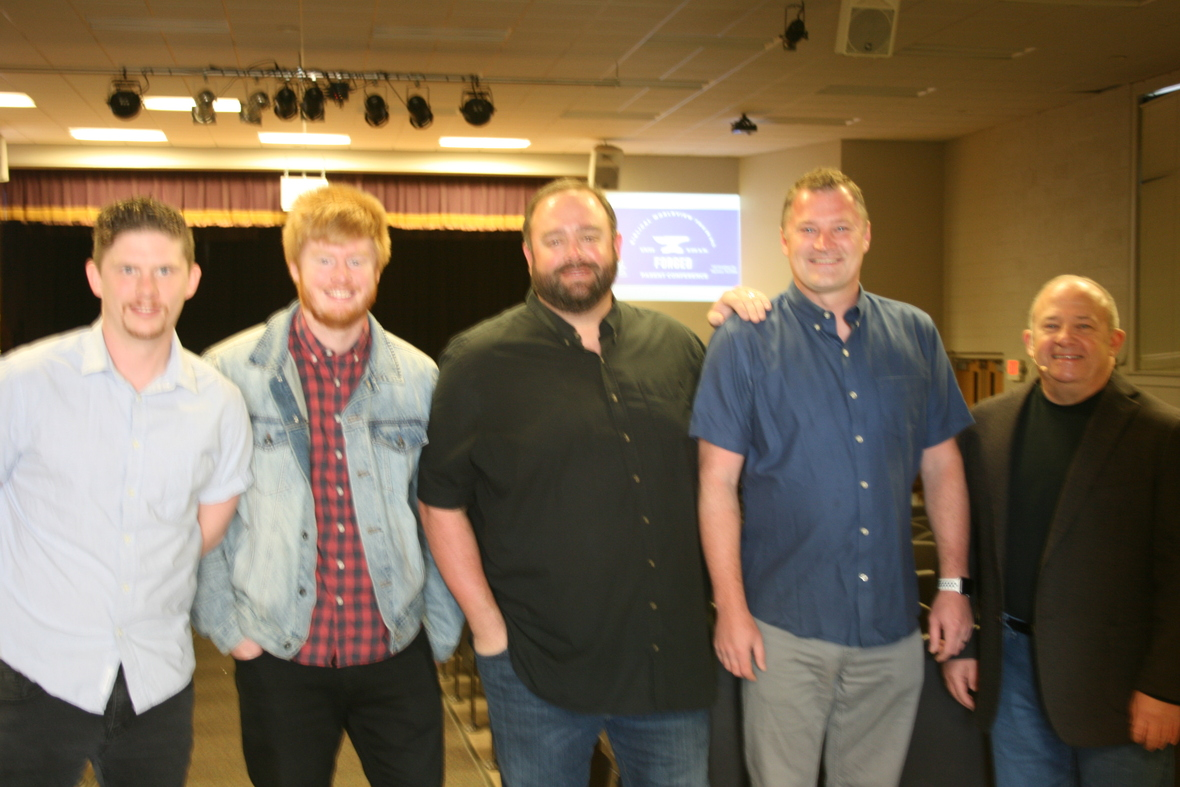# Forged Parent Conference

The Forged Conference was held on Saturday, March 5 in Woody Auditorium.
We were blessed to be led in times of worship and teaching by local pastors. Great insight and Bible based teaching was given as the speakers delved into difficult topics regarding how to lead children through trying times such as our nation currently faces. Topics such as discipleship, technology, and the importance of Biblical communication and conflict resolution were discussed.

The confernce gave parents a Biblical worldview as they face the challenges of our post-Christian society. Thank you to our speakers who gave us great insight and a charge to model Christ for our children.

As pictured above: Collin O'Neil (Worship Ministry, Sevier Heights), Matthew Gooch (Student Pastor, Sevier Heights), Anthony Kandel (Senior Pastor, Connect Church), Dr. Corey Cain (Senior Pastor, FBC Seymour, and Glenn Metts (Senior Pastor, Shiloh Baptist)

 table div table+table+table+table+table+table+table+table div table{width:100%;padding:0}table div table+table+table+table+table+table+table+table div table img{width:96.23%;padding:0;float:none}table div table+table+table+table+table+table+table+table div table td{width:100%;padding:0 1.88% 18px}/* styles */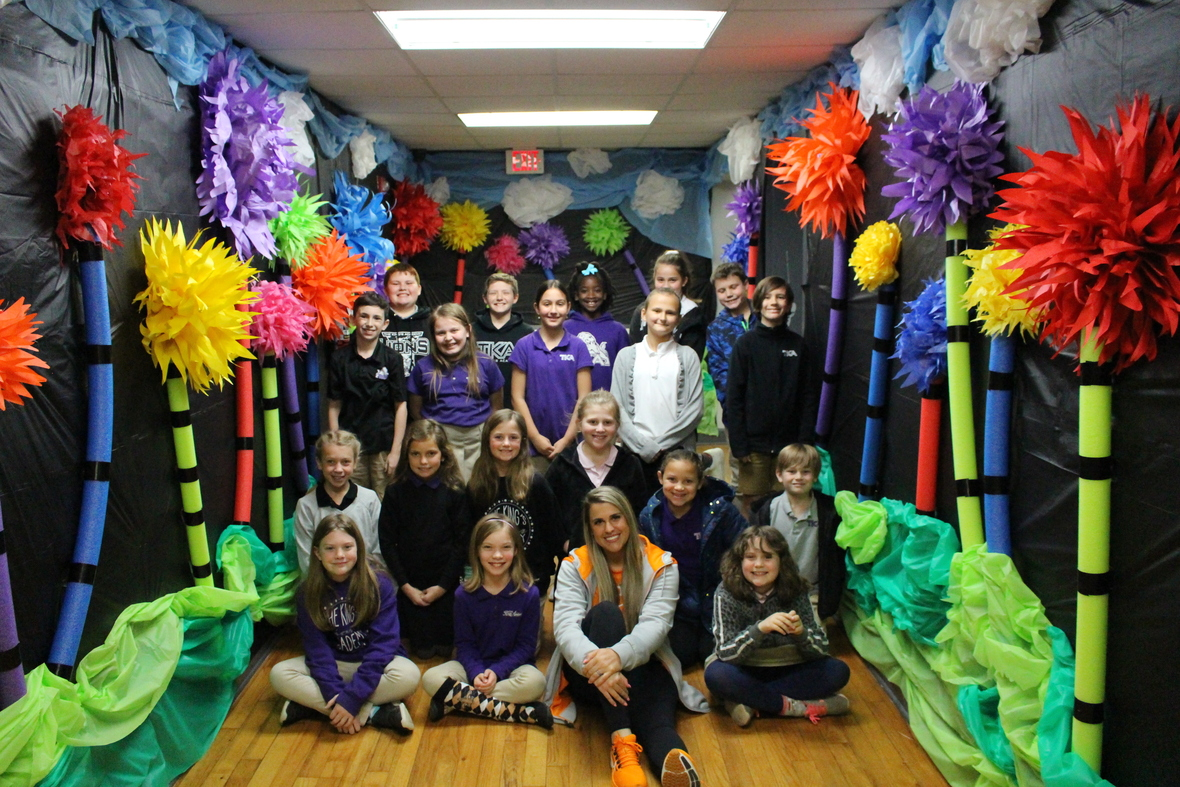Super Reader Week 2022 was a hit! We want to share our greatest appreciation to all of our Super Readers who took time out of their schedule to come read to our students. We had parents, teachers, coaches, high school students, administration, and alumni on campus last week as Super Readers to share in our celebration for reading.

 table div table+table+table+table+table+table+table+table+table+table div table{width:100%;padding:0}table div table+table+table+table+table+table+table+table+table+table div table img{width:96.23%;padding:0;float:none}table div table+table+table+table+table+table+table+table+table+table div table td{width:100%;padding:0 1.88% 18px}/* styles */This speaks volumes about our phenomenal educators and how they pour daily into our little lions. It also shows the powerful parent involvement and partnership here at TKA because for reading levels to remain at the high level they are, it takes our parents reading with their child daily to continue to hone/reinforce the skills learned during class. We thank you for your support and partnership!

Keep up the good work, little lions! Let's continue to ROAR!

 table div table+table+table+table+table+table+table+table+table+table+table+table div table{width:100%;padding:0}table div table+table+table+table+table+table+table+table+table+table+table+table div table img{width:96.23%;padding:0;float:none}table div table+table+table+table+table+table+table+table+table+table+table+table div table td{width:100%;padding:0 1.88% 18px}/* styles */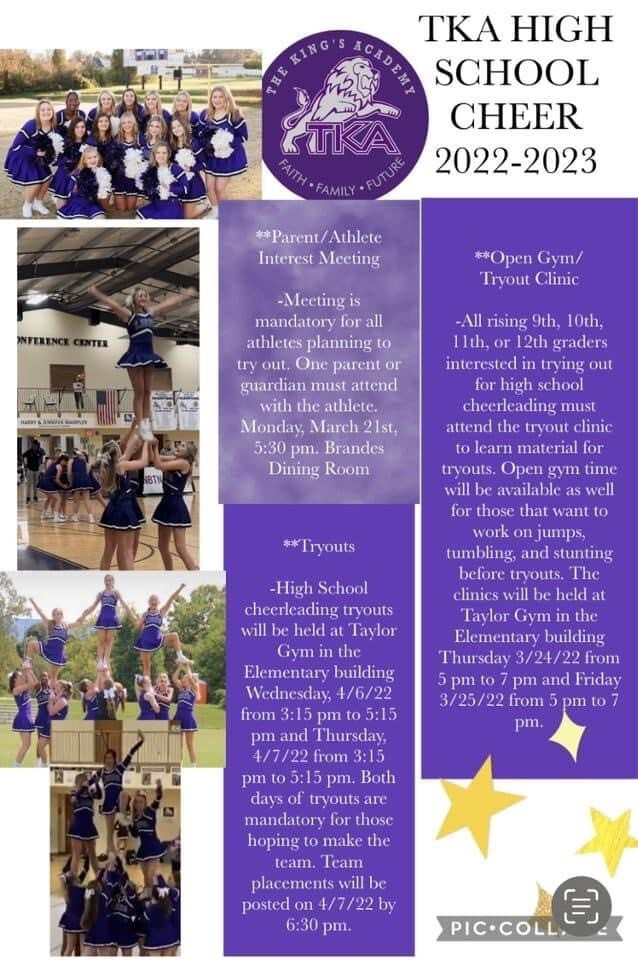table div table+table+table+table+table+table+table+table+table+table+table+table+table+table div table{width:100%;padding:0}table div table+table+table+table+table+table+table+table+table+table+table+table+table+table div table img{width:96.23%;padding:0;float:none}table div table+table+table+table+table+table+table+table+table+table+table+table+table+table div table td{width:100%;padding:0 1.88% 18px}/* styles */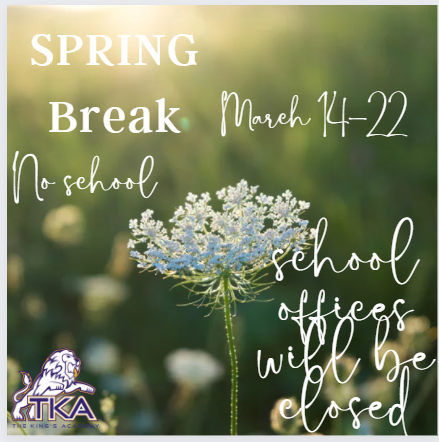# Spring Break and Teacher Workdays

Everyone be safe and enjoy your break!

 table div table+table+table+table+table+table+table+table+table+table+table+table+table+table+table+table div table{width:100%;padding:0}table div table+table+table+table+table+table+table+table+table+table+table+table+table+table+table+table div table img{width:96.23%;padding:0;float:none}table div table+table+table+table+table+table+table+table+table+table+table+table+table+table+table+table div table td{width:100%;padding:0 1.88% 18px}/* styles */table div table+table+table+table+table+table+table+table+table+table+table+table+table+table+table+table+table+table div table{width:100%;padding:0}table div table+table+table+table+table+table+table+table+table+table+table+table+table+table+table+table+table+table div table img{width:96.23%;padding:0;float:none}table div table+table+table+table+table+table+table+table+table+table+table+table+table+table+table+table+table+table div table td{width:100%;padding:0 1.88% 18px}/* styles */# PARENT CONFERENCES

Tuesday, March 22, 2022

PreK3- 12 grade parent conferences will be taking place during this day. Preschool and elementary parents need to sign up for a conference time by contacting your student's teacher.

Middle school and
High school parents can sign up for conference times by clicking here.

 table div table+table+table+table+table+table+table+table+table+table+table+table+table+table+table+table+table+table+table+table div table{width:100%;padding:0}table div table+table+table+table+table+table+table+table+table+table+table+table+table+table+table+table+table+table+table+table div table img{width:96.23%;padding:0;float:none}table div table+table+table+table+table+table+table+table+table+table+table+table+table+table+table+table+table+table+table+table div table td{width:100%;padding:0 1.88% 18px}/* styles */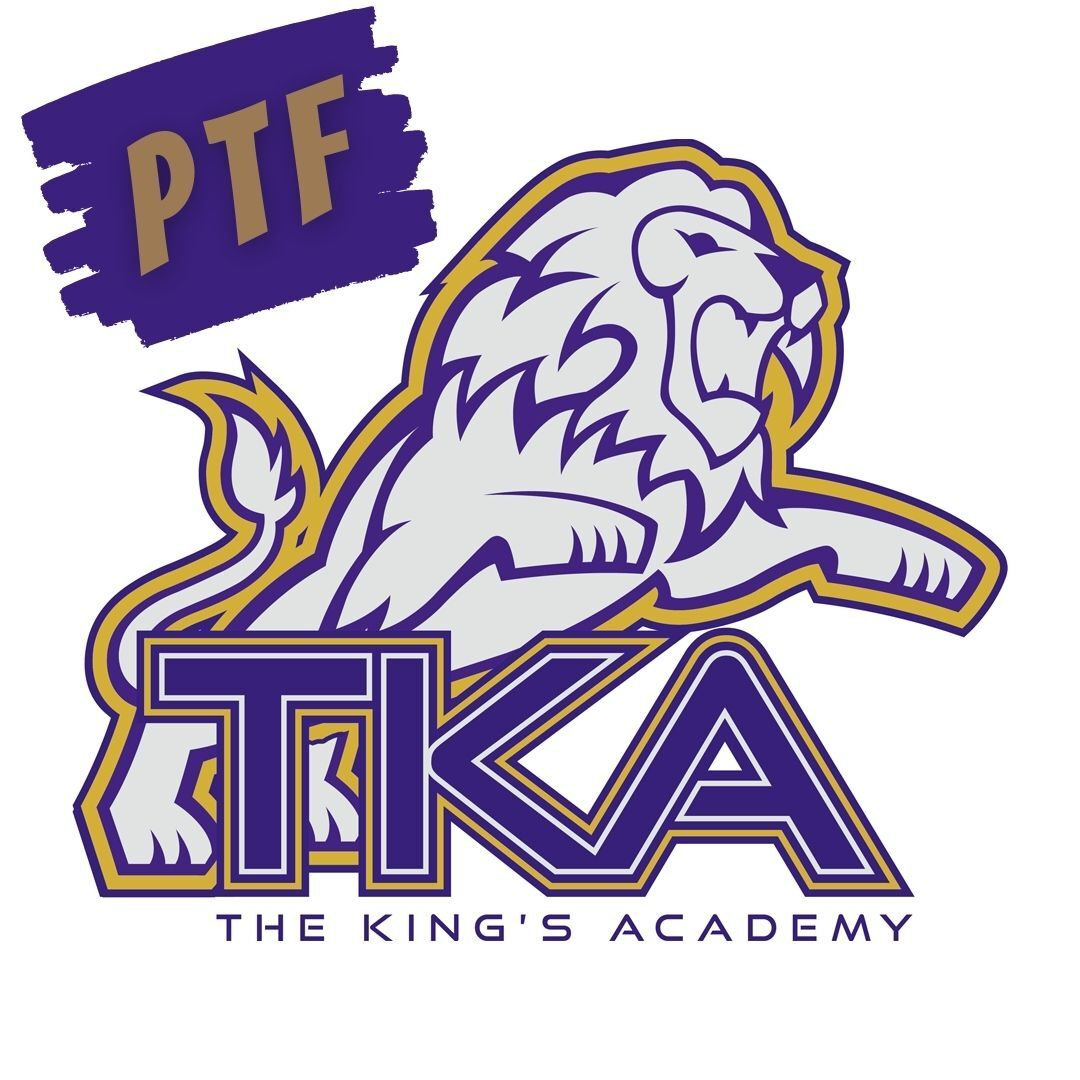# Save these Dates!

Below are the upcoming PTF meetings for the remainder of the year from 3:15 - 4:15 in Brandes:

March 31
April 28
* May 19

PTF will also host a Mother/Son kickball event on Saturday, April 30, at 12:00 p.m. More information is to come in the days ahead!

 table div table+table+table+table+table+table+table+table+table+table+table+table+table+table+table+table+table+table+table+table+table+table div table{width:100%;padding:0}table div table+table+table+table+table+table+table+table+table+table+table+table+table+table+table+table+table+table+table+table+table+table div table img{width:96.23%;padding:0;float:none}table div table+table+table+table+table+table+table+table+table+table+table+table+table+table+table+table+table+table+table+table+table+table div table td{width:100%;padding:0 1.88% 18px}/* styles */# Elementary Summer Tutoring

Dear TKA Families,

My name is Ms. Addison, and I teach third grade here at TKA. I have been a part of the TKA family for three years now, including a portion of my masters degree internship program. I am excited to announce I will be offering summer tutoring for elementary grades in reading, math, and ELA. I will be offering tutoring to better solidify skills from the grade the student will be leaving, or working on skills to prepare for the upcoming school year. The cost will be \$35/hour which can be made payable through the school. I will be working out of my classroom in the Ogle Elementary building.

Please select the dates and times from the Google from below that you are requesting to sign your student up for. After the deadline of April 8th, 2022, I will organize the requests, finalize a schedule and reach out to each family in regards to your request. Please know space is limited and not all requests will be able to be fulfilled.

 /* styles */ Thank you in advance for the opportunity to be a part of your student's education. I look forward to a fun and successful summer. Ms. Addison 3rd Grade Teacher
 table div table+table+table+table+table+table+table+table+table+table+table+table+table+table+table+table+table+table+table+table+table+table+table+table+table+table div table{width:100%;padding:0}table div table+table+table+table+table+table+table+table+table+table+table+table+table+table+table+table+table+table+table+table+table+table+table+table+table+table div table img{width:96.23%;padding:0;float:none}table div table+table+table+table+table+table+table+table+table+table+table+table+table+table+table+table+table+table+table+table+table+table+table+table+table+table div table td{width:100%;padding:0 1.88% 18px}/* styles */# Upcoming Sports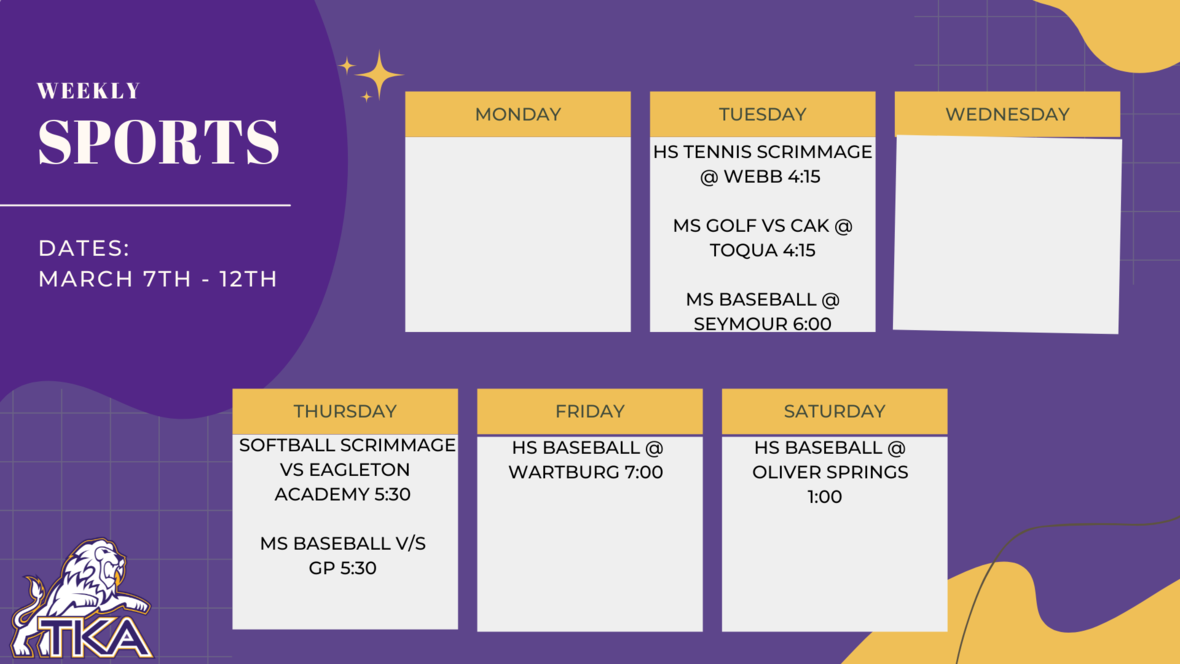table div table+table+table+table+table+table+table+table+table+table+table+table+table+table+table+table+table+table+table+table+table+table+table+table+table+table+table+table+table div table{width:100%;padding:0}table div table+table+table+table+table+table+table+table+table+table+table+table+table+table+table+table+table+table+table+table+table+table+table+table+table+table+table+table+table div table img{width:96.23%;padding:0;float:none}table div table+table+table+table+table+table+table+table+table+table+table+table+table+table+table+table+table+table+table+table+table+table+table+table+table+table+table+table+table div table td{width:100%;padding:0 1.88% 18px}/* styles */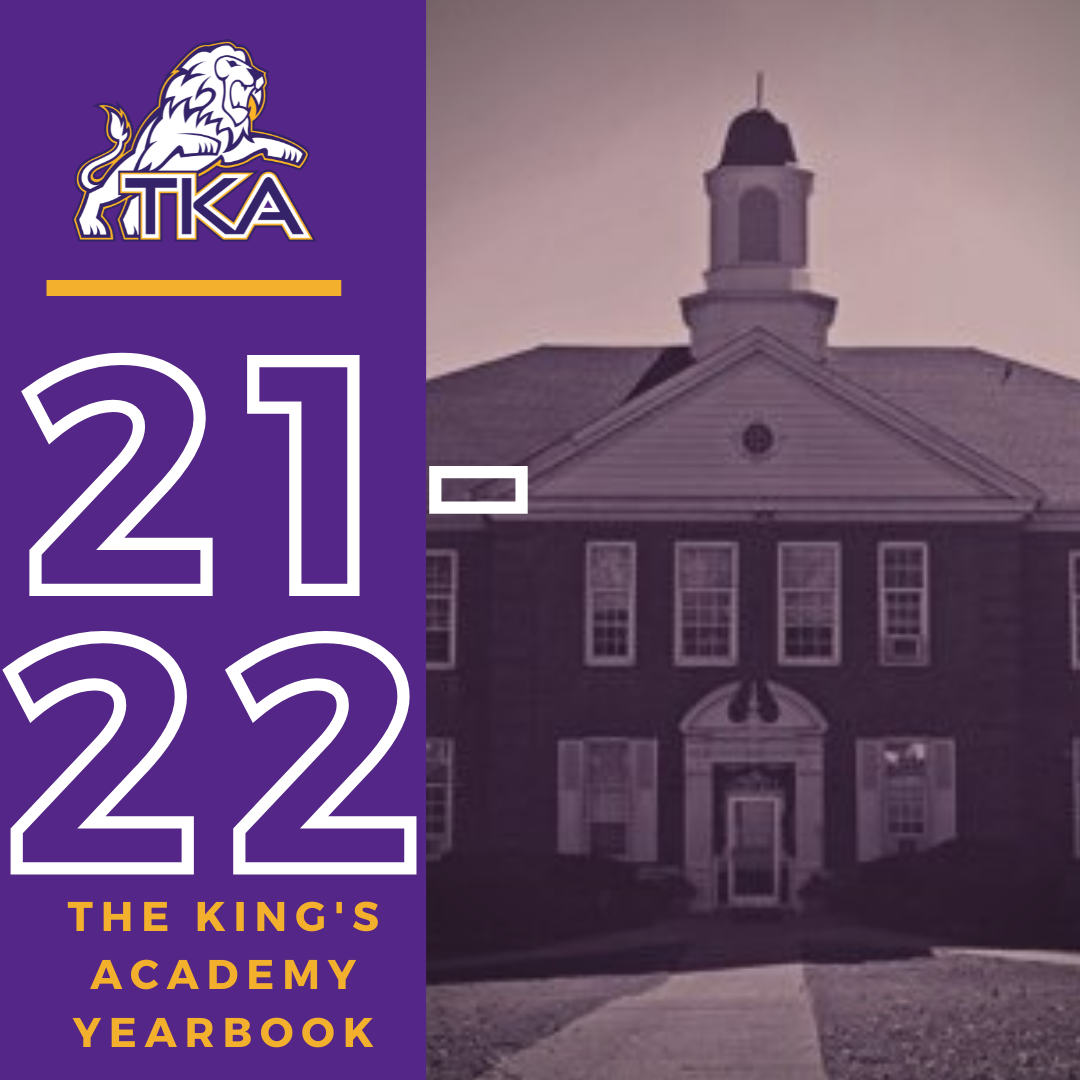# 2021-2022 Yearbook

The price for the 2021-2022 TKA yearbook is \$55. All grades, K3-12 will have the same yearbook. The slight price increase is because we are only going to have one larger all-school book.

All yearbook orders can be placed online--here is the link.Do you have a class of 2022 senior in your household?

Don't miss out on the opportunity to purchase a Senior Tribute for the 2021-2022 yearbook!

Senior Tributes are available for purchase at the bottom of the link listed here.For any questions regarding yearbooks, email Mr. Josh Smith at jsmith@thekingsacademy.net.

 table div table+table+table+table+table+table+table+table+table+table+table+table+table+table+table+table+table+table+table+table+table+table+table+table+table+table+table+table+table+table+table+table+table div table{width:100%;padding:0}table div table+table+table+table+table+table+table+table+table+table+table+table+table+table+table+table+table+table+table+table+table+table+table+table+table+table+table+table+table+table+table+table+table div table img{width:96.23%;padding:0;float:none}table div table+table+table+table+table+table+table+table+table+table+table+table+table+table+table+table+table+table+table+table+table+table+table+table+table+table+table+table+table+table+table+table+table div table td{width:100%;padding:0 1.88% 18px}/* styles *//* styles */
 table div table+table+table+table+table+table+table+table+table+table+table+table+table+table+table+table+table+table+table+table+table+table+table+table+table+table+table+table+table+table+table+table+table+table+table+table div table{width:100%;padding:0}table div table+table+table+table+table+table+table+table+table+table+table+table+table+table+table+table+table+table+table+table+table+table+table+table+table+table+table+table+table+table+table+table+table+table+table+table div table img{width:96.23%;padding:0;float:none}table div table+table+table+table+table+table+table+table+table+table+table+table+table+table+table+table+table+table+table+table+table+table+table+table+table+table+table+table+table+table+table+table+table+table+table+table div table td{width:100%;padding:0 1.88% 18px}/* styles */# Covid-19 Procedures

While we are excited to offer in-person education, we must maintain a safe learning environment for all students, faculty/staff, and additional stakeholders.

To do so, we will be working closely with the Sevier County Health Department regarding Covid-19 protocols.

 table div table+table+table+table+table+table+table+table+table+table+table+table+table+table+table+table+table+table+table+table+table+table+table+table+table+table+table+table+table+table+table+table+table+table+table+table+table+table div table{width:100%;padding:0}table div table+table+table+table+table+table+table+table+table+table+table+table+table+table+table+table+table+table+table+table+table+table+table+table+table+table+table+table+table+table+table+table+table+table+table+table+table+table div table img{width:96.23%;padding:0;float:none}table div table+table+table+table+table+table+table+table+table+table+table+table+table+table+table+table+table+table+table+table+table+table+table+table+table+table+table+table+table+table+table+table+table+table+table+table+table+table div table td{width:100%;padding:0 1.88% 18px}/* styles */table div table+table+table+table+table+table+table+table+table+table+table+table+table+table+table+table+table+table+table+table+table+table+table+table+table+table+table+table+table+table+table+table+table+table+table+table+table+table+table+table div table{width:100%;padding:0}table div table+table+table+table+table+table+table+table+table+table+table+table+table+table+table+table+table+table+table+table+table+table+table+table+table+table+table+table+table+table+table+table+table+table+table+table+table+table+table+table div table img{width:96.23%;padding:0;float:none}table div table+table+table+table+table+table+table+table+table+table+table+table+table+table+table+table+table+table+table+table+table+table+table+table+table+table+table+table+table+table+table+table+table+table+table+table+table+table+table+table div table td{width:100%;padding:0 1.88% 18px}/* styles */